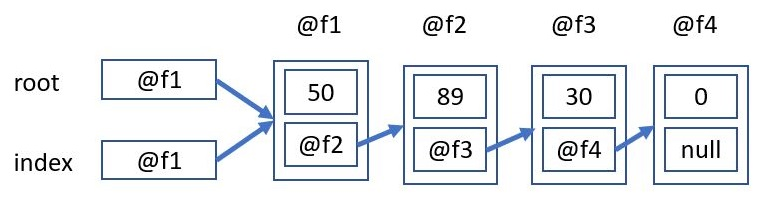# 陣列的限制

int []array=new int;
a 是一個長度為10個元素的陣列. 一經宣告, 長度就不能改變. 重點在於一開始我們怎麼知道是要宣告為 10, 或 100.

# 解決方案# 程式代碼

class Node{
int d;
Node next;
}

public class SingleLink {
public static void main(String[] args){
Node root=new Node();
Node index=root;
Scanner in=new Scanner(System.in);
int x=0;
while(true){
System.out.print("請輸入數字(-1離開) : ");
x=in.nextInt();
if(x==-1)break;
index.d=x;
index.next=new Node();
index=index.next;
}
index=root;
while(index.next!=null){
System.out.printf("%d ", index.d);
index=index.next;
}
System.out.println();
}
}
class Node{
int d;
Node next;
}

10 50 60 70 80 90